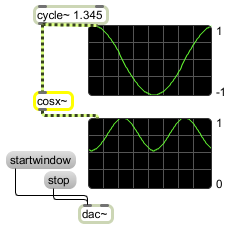# cosx~

Signal cosine function

## Description

cosx~ will take any given signal and output a signal which is the hyperbolic cosine function of each sample within the input signal, but unlike the cos~ object, whose output is based around 1 and intended for use as a lookup table with the phasor~ object, the cosx~ object is a true π based function.

None.

## Messages

 signal Output from a cosine function.

## Output

signal: The cosine of the input.

## Examplescosx~ can make your audio control signals less jumpy and more bumpy

Name Description
acos~ Signal arc-cosine function
acosh~ Signal hyperbolic arc-cosine function
asin~ Signal arc-sine function
asinh~ Signal hyperbolic arc-sine function
atan~ Signal arc-tangent function
atanh~ Signal hyperbolic arc-tangent function
atan2~ Signal arc-tangent function (two variables)
cos~ Signal cosine function (0-1 range)
cosh~ Signal hyperbolic cosine function
sinh~ Signal hyperbolic sine function
sinx~ Signal sine function
tanh~ Signal hyperbolic tangent function
tanx~ Signal tangent function